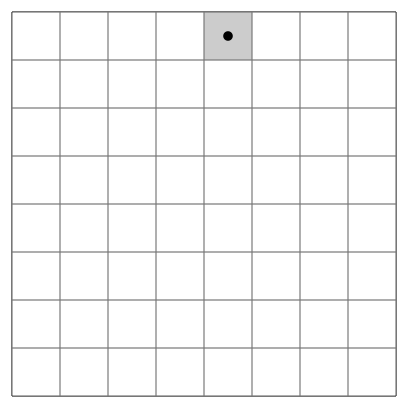# Unbounded Knapsack — Day 49(Python)

Today’s problem is another variation of the Knapsack problem. In the earlier version of the knapsack problem, we had a limited number of items. In the current version of the problem, we have an unlimited number of item counts. Let’s jump into the question.

# Unbounded Knapsack (Repetition of items allowed)

Given a knapsack weight W and a set of n items with a certain value val[i] and weight wt[i], we need to calculate the maximum amount that could make up this quantity exactly. This is different from the classical Knapsack problem, here we are allowed to use an unlimited number of instances of an item.
Examples:

`Input : W = 100       val[]  = {1, 30}       wt[] = {1, 50}Output : 100There are many ways to fill knapsack.1) 2 instances of 50 unit weight item.2) 100 instances of 1 unit weight item.3) 1 instance of 50 unit weight item and 50   instances of 1 unit weight items.We get maximum value with option 2.Input : W = 8       val[] = {10, 40, 50, 70}       wt[]  = {1, 3, 4, 5}       Output : 110 We get maximum value with one unit ofweight 5 and one unit of weight 3.`

We know the solution to the 0/1 Knapsack problem. To solve the 0/1 Knapsack problem using recursion, follow this link. In case you need to learn to solve the 0/1 Knapsack problem using memoization, follow this link. To solve the problem using the tabulated format, follow this link.

I will be solving this problem using dynamic programming. We have to make a few changes to the 0/1 Knapsack problem’s code.

Only difference between Unbounded Knapsack and 0/1 Knapsack is having unlimited instance of items. We need to make change the code such that we can have multiple instance of same item. Let us recall the code for 0/1 Knapsack.

`def knapSack(W, wt, val, n):   ‘’’    :param W: capacity of knapsack     :param wt: list containing weights    :param val: list containing corresponding values    :param n: size of lists    :return: Integer   ‘’’   # code here   dp_table = [[0 for j in range(W+1)]for i in range(len(wt)+1)]   for i in range(1,len(dp_table)):      for j in range(1, len(dp_table)):          if wt[i-1] <= j:             dp_table[i][j] = max(val[i-1]+dp_table[i-1][j-wt[i-1]],dp_table[i-1][j])          elif wt[i-1] > j:             dp_table[i][j] = dp_table[i-1][j]    return dp_table[-1][-1]`

Okay! Let us move into the Unbounded Knapsack problem.

We will need a 2D array of size weight of Knapsack + 1 and the number of items + 1.

Next, we will move to initialization. The first row and the first column is initialized by 0. It is similar to the 0/1 Knapsack problem.

Next, we will be filling the remaining cell values in our array. Each item’s weight is compared with the Knapsack’s weight. If the weight of the item is lesser than the weight the knapsack can hold, then we have 2 choices,

1. Include it in the knapsack
2. Exclude it from the knapsack.

In the unbound knapsack problem, even if we decide to include an item in the knapsack, we can still include its multiple instance. How do we code this information?

`if wt[i-1] <= j:   dp_table[i][j] = max(val[i-1]+dp_table[i][j-wt[i-1]],dp_table[i-1][j])`

Thats it! We have solved the unbound knapsack problem!

Let us look at the complete code.

`def knapSack(W, wt, val, n):   ‘’’    :param W: capacity of knapsack     :param wt: list containing weights    :param val: list containing corresponding values    :param n: size of lists    :return: Integer   ‘’’   # code here   dp_table = [[0 for j in range(W+1)]for i in range(len(wt)+1)]   for i in range(1,len(dp_table)):      for j in range(1, len(dp_table)):          if wt[i-1] <= j:             dp_table[i][j] = max(val[i-1]+dp_table[i][j-wt[i-1]],dp_table[i-1][j])          elif wt[i-1] > j:             dp_table[i][j] = dp_table[i-1][j]    return dp_table[-1][-1]`

Complexity analysis.

Time Complexity

We are creating a 2D array of size ((N+1)*(W+1)) and traversing through the entire table once. Hence the time complexity is ((N+1)*(W+1)).

Space Complexity.

We are creating a 2D array of size ((N+1)*(W+1)) and hence, the space complexity is ((N+1)*(W+1)).

--

--

--

## More from Annamariya Tharayil

Software Engineer. Find me @ www.linkedin.com/in/annamariya-jt

Love podcasts or audiobooks? Learn on the go with our new app.

## OSI model(Networking series 1)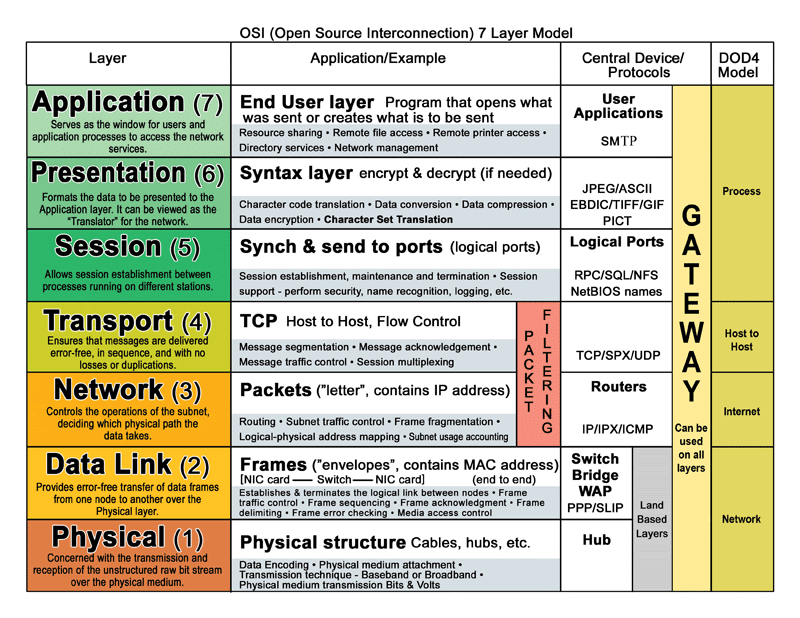## Enhanced Topologies in Cloud Pak for Watson AIOps 3.3 that Save the Day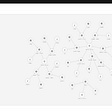## Free up Disk Space on Mac without Using Software## Cloning The New York Time## Open Source vs Closed Source — Which CMS is suitable for your industry?## A Visual Introduction to Git## Data Ingestion and Bigquery — How to load data from external sources on to BigQuery?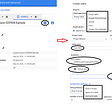## Annamariya Tharayil

Software Engineer. Find me @ www.linkedin.com/in/annamariya-jt

## Huffman coding | Greedy Algorithm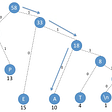## Linear Search Using Python 🐍